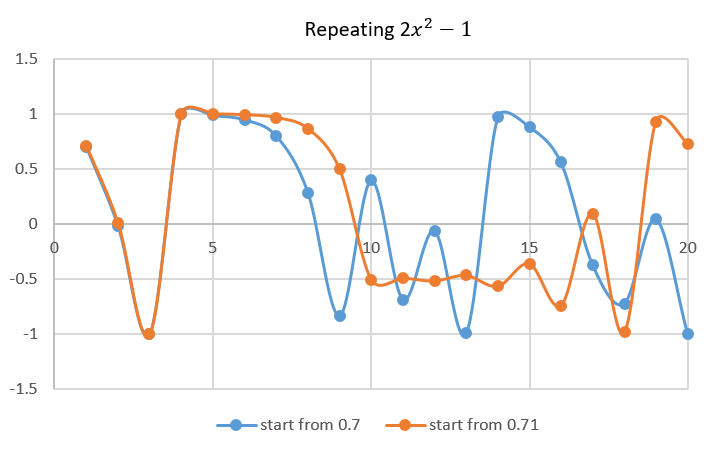Chaos implies a disorder or complex non-linear system. It manifests any little changes could probably result in a massive transformation of the system, such as a butterfly flapping in Brazil could cause a hurricane in Texas, which is well known as the butterfly effect. The traits of chaos are in many fields, including math, physics, atmospheric science, fluid dynamics, ect., which leads Chaos to a vital research topic.

There is a simple example to explain the phenomenon of chaos. There is a system that gets the results from putting numbers into the equation $$2 x^2 - 1$$ step by step. With an initial number $$x_0$$, it retrieves the answer $$x_1$$ from the equation. Then, put $$x_1$$ in the equation and get $$x_2$$, and so on repeatedly.

The following chart is the lines with initial input 0.7 and 0.71. The two lines are very close at the beginning; however, the distance between them elevates dramatically then, and you cannot ever find a pattern after step 10 - they look radically different.As you can see, only a little difference of the number could cause the line unpredictably growing up. In the real world, the system is far more complicated and colossal, making the impact of any small factors extremely significant.